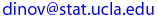Normal Approximation to Poisson(λ) Distribution

• If X ∼Poisson (λ)  ⇒  X ≈N ( μ=λ, σ=√λ), for λ>20, and the approximation improves as (the rate) λ increases.
• Poisson(100) distribution can be thought of as the sum of 100 independent Poisson(1) variables and hence may be considered approximately Normal, by the central limit theorem, so Normal( μ = rate*Size = λ*N, σ =√(λ*N)) approximates Poisson(λ*N = 1*100 = 100).
• The normal distribution is in the core of the space of all observable processes. This distributions often provides a reasonable approximation to variety of data. The Central Limit Theorem states that to the distribution of the sample average (for almost any process, even non-Normal) is normally distributed (provided the process has well defined mean and variance).
• This applet draws random samples from Poisson distribution, constructs its histogram (in blue) and shows the corresponding Normal approximation (in red). You can specify the rate (λ) of the Poisson distribution and the number of trials (N) in the dialog boxes. By changing these parameters, the shape and location of the distribution changes. This Applet gives you an opportunity to study how the approximation to the normal distribution changes when you alter the parameters of the distribution.

Last modified on by.

Ivo D. Dinov, Ph.D., Departments of Statistics and Neurology, UCLA School of Medicine